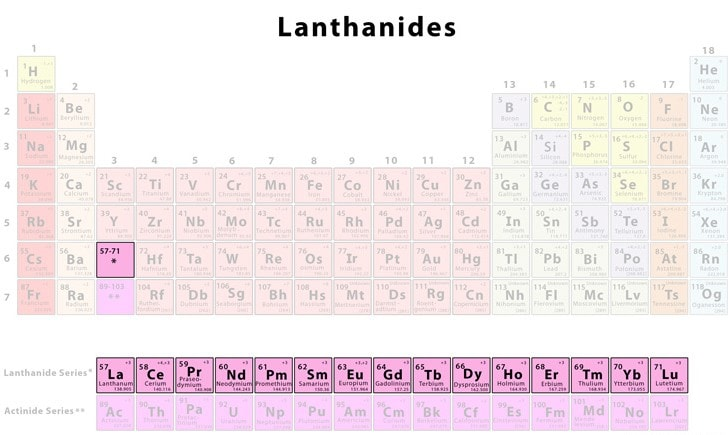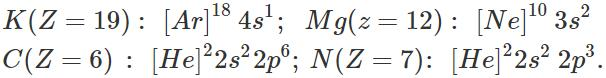Courses

# Short & Long Answer Question: Classification of Elements & Periodicity in Properties, JEE Notes | EduRev

## JEE : Short & Long Answer Question: Classification of Elements & Periodicity in Properties, JEE Notes | EduRev

The document Short & Long Answer Question: Classification of Elements & Periodicity in Properties, JEE Notes | EduRev is a part of the JEE Course Chemistry for JEE.
All you need of JEE at this link: JEE

Q.1 Why is atomic number a better basis for classification of the elements than atomic mass?

Answer: The atomic mass of the element relates to the nucleus which is present in the centre of the atom. But the properties of the elements depend upon the electronic configuration which is linked with atomic number. As the atomic number or electronic configuration atom changes, the properties of the element involved also change. Therefore, atomic number is a better basis for the classification of the elements than the atomic mass.

Q.2 Why do elements in a group show similar chemical properties?

Answer: In a group, all the elements present have the same valence shell electronic configuration of their atoms. Therefore, the elements belonging to the same group show similar chemical properties.

Q.3 Third period has eight and not eighteen elements. Explain.

Answer: No. of elements present in a particular period correspond to the electrons in the energy shell. The third period corresponds to the third energy shell which has eight electrons. Therefore, the third period has eight elements.

Q.4 Hydrogen is regarded as a rogue element in the periodic table. Discuss.

Answer: Hydrogen is regarded as a rogue element in the periodic table because till today its position is not satisfactory. Although it has been placed on the top of alkali metals on the basis of electronic con-figuration, but it is a non-metal and also resembles halogens of group 17 in many characteristics.

Q.5 Why are there fourteen elements in the lanthanoid series?

Answer: In the lanthanoid series, the electrons are filled in 4f sub-shell. Since the 4f sub-shell can have a maximum of fourteen electrons, there are fourteen elements in the lanthanoid series.Lanthanoid Series

Q.6 Why is ΔiH1 of Mg more than that of Al?

Answer: The ΔiH1 of Al is expected to be more than that of Mg because of smaller size and greater nuclear charge. But actually it is less since the electronic configuration of Mg is more symmetrical than that of Al. For details, consult Section 3.15.

Q.7 Ne and Na+ ions are isoelectronic species. Do they have same ionization enthalpies also?

Answer: No, they do not have the same ionization enthalpies because the nuclear charges are not same. Na+ion with greater nuclear charge has more ionisation enthalpy than Ne.

Q.8 The valence of representative elements is either equal to the number of valence electrons or eight minus this number. Explain.

Answer: The representative elements belonging to s and p-blocks tend to have noble gas configuration of their atoms. To achieve this, they do so either by losing the valence electrons or gaining electrons which is equal to eight minus the number of valence electrons. For example, valence of sodium (Z = 11) is one because it has one valence electron (2, 8, 1) which it loses to have the configuration of neon. Similarly, the valence of chlorine (Z = 17) is also one because it has seven valence electrons (2, 8, 7) and accepts one electron (8 - 7 = 1) to have the configuration of nearest noble gas element argon.

Q.9 Why isΔiHvalue of an element more than its ΔiH1 value?

Answer: ΔiH1 value is the energy needed to remove first electron from the gaseous atom. With the loss of the electron, the hold of the nucleus on the remaining electrons increases. Therefore, greater energy is needed to remove the second electron from the gaseous atom. The  ΔiH2 value of an element is always more than its ΔiH1 value. For detail, consult text part.

Q.10 Chlorine can be converted into chloride ion more easily as compared to fluoride ion from fluorine. Explain.

Answer: The negative electron gain enthalpy of chlorine (ΔegH=−349kJmol−1)  is more than that of fluorine (ΔegH=−328kJmol−1). This means that an atom of chlorine has a greater attraction for the outside electron than in case of fluorine. Therefore, chlorine can be converted to chloride ion more readily as compared to fluorine forming fluoride ion.

Q.11 Alkali metals do not form dipositive ions. Why?

Answer: The general, electronic configuration of the alkali metals is ns(n is valence shell). By losing the valence electron, they acquire the configuration of the nearest noble gas element and therefore, have no urge or tendency to lose another electron to form dipositive ion.

Q.12 Among the elements K, Mg, C and N which would show the maximum difference in the first and second ionization enthalpies.

Answer: The electronic configurations of the elements are:The element K is expected to show the maximum difference in ΔiH1 and ΔiH2  values. Actually, when its atom loses one electron; the cation left has the noble gas configuration. The removal of the second electron from the ion requires very high ionization enthalpy (ΔiH2).

Q.13 What would be IUPAC names and symbols for elements with atomic numbers 122, 127, 135, 149 and 150?

Answer: From the Table 3.6, page 3/11, the roots for 2, 7, 5, 9 and 0 are bi sept, pent, enn and nil respectively. Therefore, their names and symbols are : Z Name  Symbol 122 Unbibium Ubb 127 Unbiseptium Ubs 135 Untripentium  Utp 149 Unquadennium Uqe 150 Unpetnilium  Upn.

Q.14 The element 119 has not been discovered. What could be the IUPAC name and symbol for this element? On the basis of the periodic table, predict the electronic configuration of this element and also the formula of its most stable chloride and oxide.

Answer: (i) The roots for 1 and 9 are un and enn respectively. Therefore, the name for the element with Z = 119 is un + un + enn + ium = ununennium and its symbol is Uue. (ii) The maximum number of elements which can be accommodated in the present set up of the long form of the periodic table = 118 (For details refer to Q. 2, page 3/91). Since this last element would be an inert gas, therefore, the element with Z = 119 will be an alkali metal and hence its outer electronic configuration will be 8s1 or its complete electronic configuration will be 1s22s22p63s23p63d104s24p64d104f145s2 5p65d105f146s26p66d107s27p68s1. (iii) Since the element with Z = 119 will be an alkali metal, therefore, formula of its chloride will be MCl and that of its stable oxide will be bold M2O where M is the alkali metal.

Q.15 Why has the zero group been placed at the extreme right of the periodic table?

Answer: This is in accordance with their electronic configurations because each period starts with the filling of s-subshell and is completed with the filling of p-subshell of the same principal shell.

Q.16 The first ionization enthalpy of carbon atom is greater than that of boron whereas the reverse is true for the second ionization enthalpy. Explain.

Answer: E.C. of C-atom is 1s22s22p2 and E.C. of B-atom is 1s22s22p1. The first electron to be removed in both cases is from a 2p-orbital but nuclear charge of C is more than that of B. Therefore, the ΔiH1 of C is greater than that of B. After the removal of first electron, the second electron to be removed from C-atom is from a 2p-orbital whereas that from B-atom is from a 2s orbital. Since a s-orbital is more penetrating and hence is more strongly attracted by the nucleus than a p-orbital, therefore, ΔiH2 of B is higher than that of C.

Q.17 Which of the elements Na, Mg, Si and P would have the greatest difference between the first and the second ionization enthalpies. Briefly explain your answer.

Answer: Among Na, Mg, Si and P, Na is an alkali metal. It has only one electron in the valence shell, therefore, its ΔiH1 is very low. However, after removal of one electron, it acquires neon gas configuration, i.e., Na+(1s22s22p6). Therefore, its ΔiH2 is expected to be very high. Consequently, the difference in first and second ionization enthalpies would be greatest in case of Na. However, it may be noted here that in case of Mg, Si and P, although theirΔiH1 will be much higher than that of Na but their ΔiH2 will be much lower than that of Na. As a result, the difference in their respectiveΔiH1 and ΔiH2 would be much lower than that of Na.

Q.18 Arrange the following elements in the increasing order of non-metallic character : B, C, Si, N, F.

Answer: Arranging the elements into different groups and periods in order of their increasing atomic numbers, we have, 13           14           15           16           17 B             C             N             -              F -              Si             -              -              - Since the metallic character increases down the group and non-metallic character increases along a period from left to right, therefore, Si is the most metallic or the least non-metallic element. The overall increasing order of non- metallic character is: Si<B<C<N<F.

Q.19 Arrange the following ions in order of decreasing ionic radii: Li2+,He+,Be3+.

Answer: He+,Li2+and Be3+ are all isoelectronic ions. Among isoelectronic ions, ionic radius decreases as the positive charge increases. Therefore, the ionic radii decrease in the order: He+>Li2+>Be3+.

Offer running on EduRev: Apply code STAYHOME200 to get INR 200 off on our premium plan EduRev Infinity!

## Chemistry for JEE

196 videos|450 docs|369 tests

,

,

,

,

,

,

,

,

,

,

,

,

,

,

,

,

,

,

,

,

,

,

,

,

;